### Home > MC2 > Chapter 6 > Lesson 6.2.2 > Problem6-90

6-90.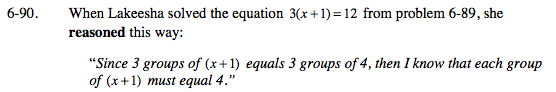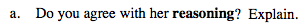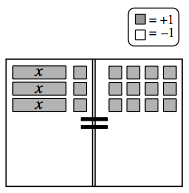Recall the Equation Mat from problem 6-89.
Each group of (x + 1) corresponds to each group of 4.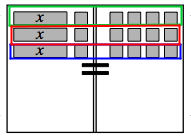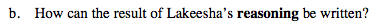x + 1 = 4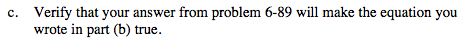Substitute your answer from problem 6-89 into the equation above and see if the equation is true.